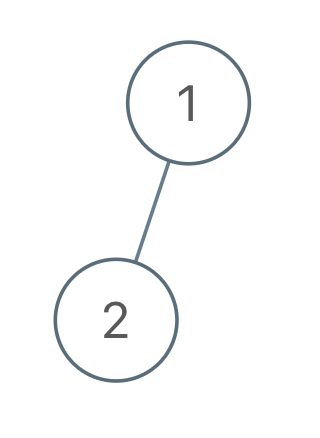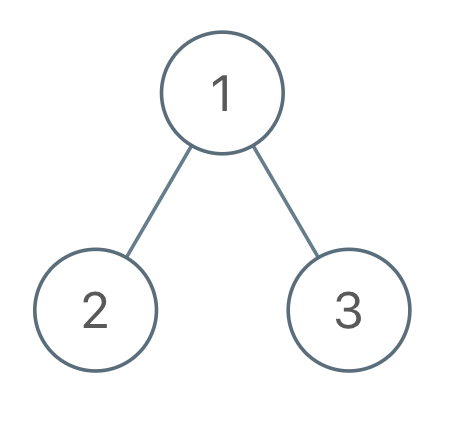## 971. Flip Binary Tree To Match Preorder Traversal

You are given the `root` of a binary tree with `n` nodes, each node has a different value from `1` to `n`. You are also given a sequence of `n` values `voyage`, reported by a preorder traversal starting from the `root`.

A node in this binary tree can be flipped by swapping its left child and its right child.

Flip the least number of nodes in the tree so that the preorder traversal of the tree matches `voyage`.

Return a list of the values of all nodes flipped. You may return the answer in any order. If we cannot flip the nodes in the tree to obtain `voyage`, return the list `[-1]`.

The preorder traversal of a node means we report the current node's value, then preorder-traverse the left child, then preorder-traverse the right child.

Example 1:```Input: root = [1,2], voyage = [2,1]
Output: [-1]
```

Example 2:```Input: root = [1,2,3], voyage = [1,3,2]
Output: 
```

Example 3:```Input: root = [1,2,3], voyage = [1,2,3]
Output: []
```

Constraints:

• The number of nodes in the tree is `n`.
• `n == voyage.length`
• `1 <= n <= 100`
• `1 <= Node.val, voyage[i] <= n`
• All the values of the tree are unique.
• All the values of `voyage` are unique.

## Rust Solution

``````struct Solution;
use rustgym_util::*;

trait Preorder {
fn preorder(&self, size: &mut usize, nodes: &mut Vec<i32>, voyage: &[i32]) -> bool;
}

fn preorder(&self, size: &mut usize, nodes: &mut Vec<i32>, voyage: &[i32]) -> bool {
if let Some(node) = self {
let node = node.borrow();
let val = node.val;
if voyage[*size] != val {
return false;
}
*size += 1;
if node.left.is_none() && node.right.is_none() {
return true;
}
if node.left.is_some() {
if node.left.as_ref().unwrap().borrow().val == voyage[*size] {
node.left.preorder(size, nodes, voyage)
&& node.right.preorder(size, nodes, voyage)
} else {
nodes.push(val);
node.right.preorder(size, nodes, voyage)
&& node.left.preorder(size, nodes, voyage)
}
} else {
node.right.preorder(size, nodes, voyage)
}
} else {
true
}
}
}

impl Solution {
fn flip_match_voyage(root: TreeLink, voyage: Vec<i32>) -> Vec<i32> {
let mut nodes = vec![];
let mut size: usize = 0;
if root.preorder(&mut size, &mut nodes, &voyage) {
nodes
} else {
vec![-1]
}
}
}

#[test]
fn test() {
let root = tree!(1, tree!(2), None);
let voyage = vec![2, 1];
let res = vec![-1];
assert_eq!(Solution::flip_match_voyage(root, voyage), res);
let root = tree!(1, tree!(2), tree!(3));
let voyage = vec![1, 3, 2];
let res = vec!;
assert_eq!(Solution::flip_match_voyage(root, voyage), res);
let root = tree!(1, tree!(2), tree!(3));
let voyage = vec![1, 2, 3];
let res: Vec<i32> = vec![];
assert_eq!(Solution::flip_match_voyage(root, voyage), res);
}
``````

Having problems with this solution? Click here to submit an issue on github.